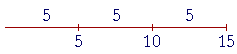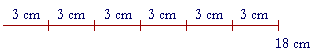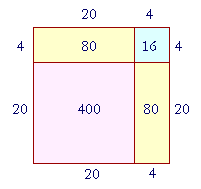S k i l l
i n
A R I T H M E T I C

Lesson 9

# THE MEANING OF MULTIPLICATION

## Mental Calculation

 1. What is the problem of "multiplication"? 3 × 5 We are to name the number that results from repeatedly adding one number, called the multiplicand, as many times as there are ones in another number, called the multiplier.That number we name is called the product of the two numbers.

3 × 5

Although we read that "3 times 5"—× is the multiplication sign—it means

5 three times.

 3 × 5 = 5 + 5 + 5 = 15.5 is the multiplicand.  We have multiplied it—repeatedly added it—as many times as there are ones in 3 --

3 = 1 + 1 + 1

-- which is the multiplier.  (In the United States we write the multiplier on the left: 3 × 5.)  The product is 15.

Multiplication, then, is an abbreviation for repeated addition.

"12 × 48" is an abbreviation for the repeated addition of 48—twelve times.

"48 × 12" is an abbreviation for the repeated addition of 12—forty-eight times.

(We will come to the most general definition of multiplication in Lesson 27.)

The numbers produced—the products—that result from repeatedly adding a number are called its multiples. (See Problem 5 at the end of the Lesson.)  Here, for example, are the first few multiples of 6:

6,  12,  18,  24,  30,  36.

In practice, of course, to name the product of 5 × 6, the student should not actually add 6 five times: 6 + 6 + 6 + 6 + 6.  That would not be skillful.  Skill in multiplication depends on knowing the multiplication table—which is a table of repeated additions.  Not only is skill in written calculation based on knowing that table, it is the basis of mental calculation, as well.  In this Lesson we continue to emphasize problems that do not require a calculator—problems that an educated person should not have to write down.

For many problems, mental calculation has always been the quickest and the most practical.

Example 1.   A case of fruit juice contains 9 bottles.  How many bottles are there in 4 cases?

 Answer.    4 × 9 bottles = 9 + 9 + 9 + 9 = 36 bottles.

This illustrates that the product will be the same kind of quantity as the multiplicand; in this case, bottles. The multiplier (4) will be a pure number.  It indicates how many times to add the multiplicand.

The student of course should know that 4 × 9 = 36The order property of multiplication

a)  What multiplication does this illustrate?Answer.   3 × 6 cm = 18 cm.  6 cm is the multiplicand.  It is being repeatedly added three times.

b)  What multiplication does this illustrate?Answer.   6 × 3 cm = 18 cm.  3 cm is the multiplicand.  It is being repeatedly added six times.

This illustrates the order property of multiplication.  If we add 3 cm six times, we will get the same number as when we add 6 cm three times.

6 × 3 cm = 3 × 6 cm.

Now, 6 and 3 are small numbers, and we know that

6 × 3 = 3 × 6 = 18.

But consider

12 × 48  and  48 × 12.

If we add 48 twelve times, is it obvious that we will get the same number as when we add 12 forty-eight times?

Although it is not obvious, we can prove that it is true—we can see it. And it has nothing to do with knowing the product of 12 × 48.

It will be simpler however to see that

3 × 6 cm = 6 × 3 cm

—and without saying that 3 × 6 = 18.

Here again is 3 × 6 cm.Do you see 6 × 3 cm ?

Look at the 1st cm in each 6.  Those are 3 cm.  Now look at the 2nd cm.  There's another 3.  And so on, up to the 6th in each group. There are the 6 × 3 cm.  And it was not necessary to name the product.

For another look at this, see Lesson 10.

In algebra, "multiplication" is not defined, and therefore algebra cannot prove the basic properties of multiplication. They are stated as laws or axioms. But in arithmetic, we define multiplication, and therefore we can prove its properties. We should prove its properties; specifically, we can prove the order property. And it is only because it is true in arithmetic, that in algebra what is called the commutative law will apply to arithmetic.

See Appendix 4, Two Theorems.

We now turn our attention to certain skills.

The order of any factors

When numbers are multiplied, they are called the factors of the product.

2 × 3 × 5

The factors are 2, 3, and 5.

The order of any number of factors does not matter.

2 × 3 × 5 = 5 × 2 × 3 = 5 × 3 × 2

And so on.  If we were to do the multiplications from left to right, then in each case we will get 30.  Therefore we may group factors as we please.

Example 2.   Multiply as easily as you can:  7 × 2 × 9 × 5

Solution.   Take advantage of factors that produce a multiple of 10:

7 × 2 × 9 × 5 = (2 × 5) × (7 × 9) = 10 × 63 = 630.

(Lesson 4.)

Here is another essential skill:

 2. How do we multiply whole numbers that end in 0's? 200 × 30 Ignore those final 0's and multiply the numbers that remain. Then, put back all the 0's that you ignored.

Example 3.   200 × 30 = 6000.

Ignore the final 0's and simply multiply

2 × 3 = 6.

Now we ignored a total of three 0's.  Therefore we must put back those three 0's:

200 × 30 = 6000.

When we ignore the 0's, we are just waiting to multiply by the power of 10 that has those 0's. (Lesson 4.)

 200 × 30 = 2 × 100 × 3 × 10 = 2 × 3 × 100 × 10 = 6 × 1000 = 6000.

Or, when we ignore final 0's, we could say that we have divided by a power of 10. (Lesson 2, Question 10.)
Therefore to compensate and name the right answer, we must multiply by that power—we must add on the same number of 0's. For, a number does not change if we divide it and then multiply by the same number. (Lesson 11.)

Example 4.    9 × 20 = 180.

Example 5.   Multiply  3 × \$.40  mentally.

Answer.  Ignore the decimal point for the moment, and we have

3 × 40 = 120.

Now put back those two decimal digits:

3 × \$.40 = \$1.20

(We will explain in the next Lesson about ignoring decimal points and then replacing them. After all, we can only do arithmetic with whole numbers, anyway)

Example 6.   7 × \$.80 = \$5.60

Decomposing the multiplier

Since multiplication is repeated addition, we can often go from what we know to what we do not know.  Say, for example, that you do not know 12 × 8.  But Twelve 8's are made up ofTen 8's are 80,

Two 8's are 16.

Therefore,

Twelve 8's are 80 + 16 = 96.

In this way, we "repeatedly added" Twelve 8's.  We decomposed them—broke them up—into

Ten + Two.

3.   How do we multiply mentally by decomposing the multiplier?
 42 × 8 = 40 × 8  +  2 × 8 = 320 + 16 = 336.

Decompose the multiplier mentally into its units, typically into its tens and ones. Multiply by the number of tens, then by the number of ones, and add those partial products.

Since it is true in arithmetic that we may multiply by decomposing the multiplier, the right-hand distributive law of algebra may be applied to arithmetic.

Example 7.   Multiply  16 × 5  mentally by decomposing 16 into its tens and ones.

 Answer.   Sixteen 5's are equal to Ten 5's  +  Six 5's: 50 + 30 = 80.

Example 8.   Calculate  34 × 6  mentally by decomposing 34.

Solution.   34 × 6  =  30 × 6  +  4 × 6  =  180 + 24  =  204.

"Thirty-four 6's are equal to Thirty 6's plus four 6's."

Example 9.  Multiplying by 11.    11 × 45.

Multiplication by 11 is particularly easy because 11 is 10 + 1.

11 × 45 therefore is  10 × 45  +  1 × 45:

450 + 45 = 495.

In other words, to multiply by 11, simply add 10 times the number to the number.

Example 10.   11 × 6.2 = 62 + 6.2 = 68.2

To do this, or any of these, problems in writing ---- is nothing more than a written trick to get the right answer.

Example 11.   How much is 13 × 12?

Answer.  If you know that

12 × 12 = 144,

then 13 × 12 is simply one more 12:

13 × 12 = 144 + 12 = 156.

Or, we could count the 12's like this:

 Thirteen 12's =  Ten 12's + Three 12's =  120 + 36 =  156.

How to square a number mentally

A square figure is a four-sided figure in which all the sides are equal, andall the angles are right angles.

To "square" a number means to multiply it by itself.

"9 squared" = 9 × 9 = 81.

To find the area of a square figure—that is, the space enclosed by the four straight lines—square the length of a side. (See Lesson 10.)  If each side were measured by number 6, for example, then the area would be measured by 6 × 6 = 36.

Now, say that we want to square a two-digit number such as 24, mentally.  The following geometrical arithmetic illustrates how.

Look at this square figure in which each side is 24.Then the area of that square will be 24 × 24.  But we can break up 24 into 20 + 4.  Therefore the entire square will be composed of the following:

The square of 20, which is 400.

Two rectangles, each with area 20 × 4 = 80.

And the square of 4, which is 16.

In other words, the square of  20 + 4  is equal to

The square of 20, plus

Two times 20 × 4, plus

The square of 4.

400 + 160 + 16 = 576.

This is not a difficult mental calculation.

Example 12.   Square 52.

Solution.   52 = 50 + 2.

The square of  50 + 2  is equal to

The square of 50, plus

Two times 50 × 2, plus

The square of 2.

2500 + 200 + 4 = 2704.

Problem 1.   Square 35.

To see the answer, pass your mouse over the colored area.
To cover the answer again, click "Refresh" ("Reload").
Do the problem yourself first!

The square of  30 + 5  is equal to:

The square of 30  +  Two times 30 × 5  +  The square of 5.

900 + 300 + 25 = 1225.

Example 13.   Square 48.

Solution.   Rather than square 40 + 8, it is simpler to square 50 − 2.

The square of 50 − 2 equals

The square of 50, minus

Two times 50 × 2, plus

The square of 2.

2500 − 200 + 4 = 2304.The entire square is the square of 50.  It is composed of the square of 48, plus two rectangles, 50 × 2—which we must subtract.  But we should not subtract the square of 2 twice.  Therefore we add it at the end.

Problem 2.   Square 39.

The square of  40 − 1  is equal to:

The square of 40  −  Two times 40 × 1  +  The square of 1.

1600 − 80 + 1 = 1521.

At this point, please "turn" the page and do some Problems.

or

Continue on to the Section 2: